# Learn Embedded Programming [Step by Step]

## Embedded Programming Tutorials for Beginners with Step By Step Guide.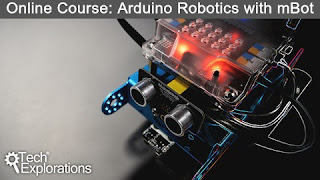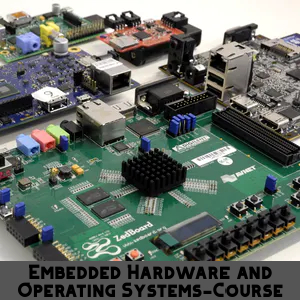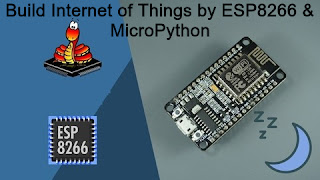## IR Controlled LED Switching : 5th & LAST PART

I have to make it quick and I think we have got all required information. I ain't going to describe source code. You have to help yourself. It has become already too long.
I can honestly say that I tried my best. Today I am going to finish this series and it's the last part.

So first of all, take a look at calculation part.

## Final Calculation :

To identify each bit uniquely, we will use another calculation method. You may use as you want. I ain't going to use millisecond. You can use millisecond or microsecond if you want.

If you want to use millisecond to measure pulse, then for logical 0 you get (562us_on+562us_off)=1.124ms. and for logical 1 you get (562us_on+1687us_off)=2.249ms.

You know, for each task  microcontroller needs time. That's why reading can be varied from calculation. So if you use integer number, you will get 1ms for logical 0 and 2ms for 1. By applying this you may successfully able to make it. Alright.

#### Now I am going to show about my method that was used on my project:

we know that , 1secon means=750000 pulses. Alright.
So, 1000ms means=750000 pulses and 1000000us means=750000 pulses.

Now, 1000000us means=750000 pulse
and   1us     ,,  = (750000/1000000)=0.75 pulses
and  562us   ,,   =(0.75*562)=421.5=421 pulses

Again,
So, 1000000us means=750000 pulse
and   1us     ,,  = (750000/1000000)=0.75 pulses
and  1687us   ,,   =(0.75*1687)=1265.25=1265 pulses

#### So, for logical 1 :

(562+1687) or (421.5+1265.75)=1686 pulses

Now, we know 255 pulses means 1 interrupt(timer overflow) or Count=1.
So ,                      1    ,,     ,,    (1/255)
So ,                    1686   ,,     ,,   (1686/255)=6.62

So, we can say 6.62 interrupts means logical 1. In coding we I will use 5 or 6 or 7 to identify logical 1 in proteus.

### So, for logical 0 :

(562+562) or (421.5+421.5)=843 pulses

Now, we know 255 pulses means 1 interrupt
(timer overflow) or Count=1.
So ,          1    ,,     ,,  (1/255) or count=(1/255)
so ,        843   ,,     ,,   (843/255)=3.31 or count=3

So, we can say 3.31 interrupts means logical 0. In coding we I will use 2 or 3 or 4 to identify logical 0 in proteus.
By using this technique, from count variable we can identify logical 1 or logical 0.

## ***Transmitter Section(Important):

In source code of transmitter , 4 commands are used .

#define command_2 0x81 or 10000001
#define command_3 0x42 or 01000010
#define command_4 0xC3 or 11000011
#define command_5 0x24 or 00100100

Here blue color represents 4bit command and red color represents reverse order of the main 4bit command (blue colored).To avoid noise and to achieve clear signal we need this.

Consider that we have main 4bit command 0001.So the reverse command should be 1000 command .Now we have to attach these and that will be 10000001. Finally we get 8bit command in which a main command and a reverse of that command are saved .

### Now We need some knowledge about PWM(Pulse Width Modulation)

We will use PWM to generate 36KHz pulse and that's why we will connect positive(+) pin of Transmitter unit  Microcontroller's  CPP1 pin . Because using that pin of Microcontroller will generate 36KHz pulse .

PWM1_Init(36000); will set up the CPP1 pin for PWM .

PWM1_Set_Duty(127);

set_duty(127) is used to providing same for both ON and OFF .That means (T/2) time it will ON and (T/2) time it will OFF .If we use set_duty(255) , then total Time period (T) will be remain ON. If we use set_duty(0) , then total Time period (T) will be remain OFF.IR (Infrared) Remote Controlled Communication Between Two Microcontroller -Step By Step Tutorial : LAST PART ( END )IR (Infrared) Remote Controlled Communication Between Two Microcontroller -Step By Step Tutorial : LAST PART ( END )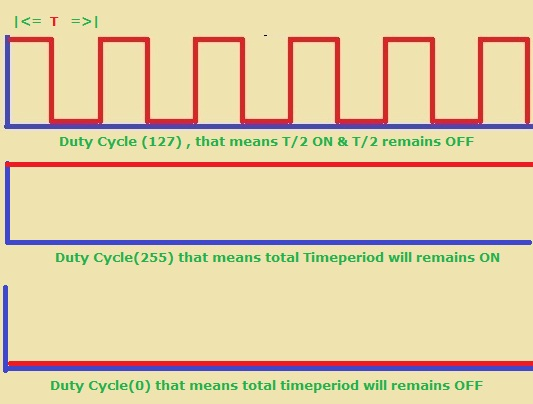IR (Infrared) Remote Controlled Communication Between Two Microcontroller -Step By Step Tutorial : LAST PART ( END )

### Again look at another picture given below

Here in if statement , an AND operation performed between Command and 0x01 . We are using this to identify each bit of data command.
Suppose , our command is 10000001 and 0x01=00000001.So ,when an AND operation performs the result will be like this.Shifting Operation in ProgrammingShifting Operation in Programming

#### So, if result is 0 ,then the related bit is 0 and delay should be 562us.

Here this part of code is comparing that Data signal commands received in receiver unit are correct .

``````   if(val==val){
txt=val;
}
if(val==val){
txt=val;
}
if(val==val){
txt=val;
}
if(val==val){
txt=val;
}
``````

## ``````  if(txt=='0' && txt=='0' && txt=='0' && txt=='1') { PORTB.F6=1; PORTB.F5=0; PORTB.F4=0; PORTB.F3=0; } ``````

When microcontroller understands about the 4bit command "0001" , consequently it turns ON RB6 pin or First LED .

## Transmitter:

``````

#define command_1 0xFF
#define command_2 0x81
#define command_3 0x42
#define command_4 0xC3
#define command_5 0x24
unsigned int i=0,j=0;
void repeat(){
PWM1_Start();
delay_us(8000);
PWM1_Stop();
delay_us(8000);
}
send_data_byte(unsigned char dattt){
PWM1_init(36000);
PWM1_Set_Duty(127);
for(j=0;j<8;j++){
PWM1_Start();
delay_us(562);
PWM1_Stop();
if(dattt & 0x01){
delay_us(1687);
dattt=dattt>>1;
}
else
{
delay_us(562);
dattt=dattt>>1;
}
}
PWM1_Stop();
}
void send_data_command(unsigned char dat){
PWM1_init(36000);
PWM1_Set_Duty(127);
PWM1_Start();
delay_us(9000);
PWM1_Stop();
delay_us(4500);
send_data_byte(dat);
repeat();
}
void main() {
CMCON=7; // to disable comparator
TRISA.F0=1;
TRISA.F1=1;
TRISA.F2=1;
TRISA.F3=1;
TRISC.F2=0;

PORTC.F2=0;
while(1){
if(PORTA.F0==0){
send_data_command(command_2);
while(PORTA.F0==0){
repeat();
}
}
if(PORTA.F1==0){
send_data_command(command_3);
while(PORTA.F1==0){
repeat();
}
}
if(PORTA.F2==0){
send_data_command(command_4);
while(PORTA.F2==0){
repeat();
}
}
if(PORTA.F3==0){
send_data_command(command_5);
while(PORTA.F3==0){
repeat();
}
}
}
}

``````

``````

unsigned int count=0,sgnal=0;
int i=7,j=0,xr=0;
char txt[]="........";
char val[]="........";
void timer_routin(){
count++;
if(count>0xFF){
count=0;
TMR0L=0;
}
}

void interrupt()
{
if(INTCON.TMR0IF){
timer_routin();
INTCON.TMR0IF=0;
}
if(INTCON.INT0IF){

xr=count;
count=0;
TMR0L=0;
if(i<0){ i=7; }
if(xr==3){ // you may use if(xr==3``````||xr==4||xr==2){...}
val[i]='0';
i--;
if(i<0){ i=7; }
}
else if(xr==6){  ``````// you may use if(xr==5||xr==6||xr==7){...}
val[i]='1';
i--;
if(i<0){ i=7; }
}
else{
/// For start signal or Repeat signal , i=0 and start to count
TMR0L=0;
i=7;
count=0;
}
INTCON.INT0IF=0;
}
}
void main()
{
CMCON=7;
TRISB=0x01;
PORTB.F6=0;
PORTB.F5=0;
PORTB.F4=0;
PORTB.F3=0;
T0CON=0xC1;
TMR0L=0;
INTCON.GIE=1;
INTCON.PEIE=1;
INTCON.TMR0IE=1;
INTCON.INT0IE=1;
INTCON.TMR0IF=0;
INTCON.INT0IF=0;
INTCON2.RBPU=1; // DISABLE PULUP REGISTER
INTCON2.INTEDG0=0;
while(1){
if(val==val){
txt=val;
}
if(val==val){
txt=val;
}
if(val==val){
txt=val;
}
if(val==val){
txt=val;
}
if(txt=='0' && txt=='0' && txt=='0' && txt=='1') {
PORTB.F6=1;
PORTB.F5=0;
PORTB.F4=0;
PORTB.F3=0;
}
if(txt=='0' && txt=='0' && txt=='1' && txt=='0') {
PORTB.F6=0;
PORTB.F5=1;
PORTB.F4=0;
PORTB.F3=0;
}
if(txt=='0' && txt=='0' && txt=='1' && txt=='1') {
PORTB.F6=0;
PORTB.F5=0;
PORTB.F4=1;
PORTB.F3=0;
}
if(txt=='0' && txt=='1' && txt=='0' && txt=='0') {
PORTB.F6=0;
PORTB.F5=0;
PORTB.F4=0;
PORTB.F3=1;
}
}
}

``````

## Time Calculation:Part-4

In this part we will connect a push button with microcontroller which will create an external interrupt and we will calculate the time duration of that interrupt .

## Calculation :

Now , we need to know how to calculate duration time of an event .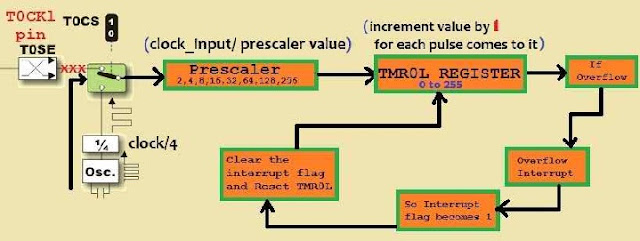IR (Infrared) Remote Controlled Communication Between Two Microcontroller -Step By Step Tutorial : Part-4

First, we  know that clock frequency will be divided by 4 and secondly, it will go to prescalar. Finally, clock goes to timer register.
So, if we use 12MHz clock and 1:4 prescalar. First we will get system clock (12/4)=3MHz clock or (12000000/4)=3000000Hz clock. Secondly it goes to prescalar and divided by 4 again. So we get (3000000/4)=750000Hz clock.
We know that for each pulse TMR0L(Timer0) register is incremented by one. So when 750000 Hz clock successfully go to timer0 register and the TMR0L register increments for 750000 times. Is it clear? We have a problem here, our TMR0L register can only count from 0 to 255.
As we need to count 750000 or greater than 750000. We have to walk in another way. We will set an integer variable count which will be incremented for each overflow interrupt. That means, when TMR0L counts 255 and overflow interrupt occurs. So it gets reset and count is incremented by one. Actually when overflow interrupt occurs, it increases the value of count variable by one. That means count=count+1;

Now consider an External Interrupt situation on RB0 pin where count=3 and TMR0L(8bit Register)=123.

How many pulses has been passed on that External Interrupt situation ?
Total pulses will be [On that situation]
tp = (count * 255) + TMR0L;

Think about that, We are using 12MHz clock and finally we get 750000Hz clock from 12MHz clock.

#### So , What is the meaning of this 750000 Hz clock frequency ?

It means, when 750000 pulses have been received, time is 1 second. We read the definition of frequency in physics. So the Time period t =(1/f);  The time required for one pulse.

### Right Now, we can say that :

tp=(count * 255)+ TMR0L ;

750000 total pulses means = 1 second

so   1    ,,     ,,    ,,       =  (1/750000)second.
so  tp    ,,     ,,    ,,   = (tp / 750000)second.
or [ (tp / 750000)*1000] miliseconds
or [ (tp / 750000)*1000000] microseconds

Using this method we can measure time duration of the interrupt.

## MikroC Code of the Project :

``````

sbit LCD_RS at RB7_bit;
sbit LCD_EN at RB6_bit;
sbit LCD_D4 at RB5_bit;
sbit LCD_D5 at RB4_bit;
sbit LCD_D6 at RB3_bit;
sbit LCD_D7 at RB2_bit;
sbit LCD_RS_Direction at TRISB7_bit;
sbit LCD_EN_Direction at TRISB6_bit;
sbit LCD_D4_Direction at TRISB5_bit;
sbit LCD_D5_Direction at TRISB4_bit;
sbit LCD_D6_Direction at TRISB3_bit;
sbit LCD_D7_Direction at TRISB2_bit;
// End LCD module connections
unsigned int count=0,sgnal=0;
int chk=7,j=0,tmr=0,cnt=0;
float tp=0.00, tr=0.00;
char txt[]="    ";
char tmrl[]="         ";
void interrupt()
{
if(INTCON.TMR0IF==1){ // checking if overflow interrupt occurs
PORTC=0x00;
INTCON.TMR0IF=0;  // clearing the overflow intrrupt flag bit
count=count+1;
if(count>=0xFF){
TMR0L=0; // clearing the timer register
count=0; // clearing count
}
}
if(INTCON.INT0IF==1){  // checking if external interrupt occur
INTCON.INT0IF=0;  // clearing the external intrrupt flag bit
// so it will be wise , to reset timer before checking the interrupt flag
cnt=count;
tmr=TMR0L;
tp=0.00;
tr=0.00;
TMR0L=0; // clearing the timer register
count=0; // clearing count
tr=(cnt * 255) + tmr ;
tp= tr/750000;
tp=tp*1000; // sec to mili second
sgnal=tp;
}
}

void main()
{
CMCON=7;
TRISC=0x00;
Lcd_Init();
Lcd_Cmd(_LCD_CLEAR);        // Clear display
Lcd_Cmd(_LCD_CURSOR_OFF);
INTCON.GIE=1;
INTCON.PEIE=1;
INTCON.INT0IE=1;
INTCON.INT0IF=0;
INTCON2.RBPU=1; // DISABLE PULUP REGISTER
INTCON2.INTEDG0=0;
T0CON=0xC1;  // setting up the timer0
INTCON.TMR0IE=1; // enabling timer overflow interrupt
while(1){
PORTC=0xFF;
inttostr(sgnal,txt);
Lcd_Out(1,1,"Time in milisec");
Lcd_Out(2,1,txt);
}
}

``````

## Proteus Circuit of the Project :

### IR (Infrared) Remote Controlled Communication Between Two Microcontroller -Step By Step Tutorial : Part-4

Note: Perhaps, we can measure the highest time: probably it is [{(255*255)+255}/750000]*1000=87.04 milliseconds. So we can measure the accurate time for 87 milliseconds probably. Actually Push button is not perfect for this. You may use another variable to increase measuring capacity.You may send pulses having time period less than 87 milliseconds on RB0 pin.  I am suggesting you provide signal on RB0 pin from another microcontroller using PWM. It will be good for you! Thank You.

## Timer :Part-3

Today I will discuss about Timer. It's very important part of microcontroller . Basically Timer is used to measuring time. Microcontroller performs time related operations using timer . As we need to measure the time of each falling edge of IR-Signal .In this tutorial we will use timer0 of pic18f2550 and we will use 8bit mode. You may use 16bit mode, but first time skip complexity. Both TMROL and TMROH registers works with 16bit mode.
PIC 18f2550 microcontroller has 4 timer modules. Timer0,Timer1,Timer2 and Timer3 . I will only describe about Timer0 and i will try to make it easy so that you can make it by yourself .

Generally Timer0 is controlled by T0CON register of pic18f2550 and TMR0L is Timer0 register low byte . In 16bit mode both TMR0L(8bit) and TMR0H(8bit) works. But in 8bit mode we can use only TMR0L. This timer0 register is incremented for each pulse comes to it and can count from 0 to 255 (0xFF).

Now we need to take a look about Timer0's control register and some necessary information . Next we will see how timer works and that will be good for us .

### Read all information carefully provided on these images!!IR (Infrared) Remote Controlled Communication Between Two Microcontroller -Step By Step Tutorial : Part-3IR (Infrared) Remote Controlled Communication Between Two Microcontroller -Step By Step Tutorial : Part-3IR (Infrared) Remote Controlled Communication Between Two Microcontroller -Step By Step Tutorial : Part-3IR (Infrared) Remote Controlled Communication Between Two Microcontroller -Step By Step Tutorial : Part-3

Just keep in your mind , all those given in previous steps.We will need those in source code .

#### Now we will see how timer works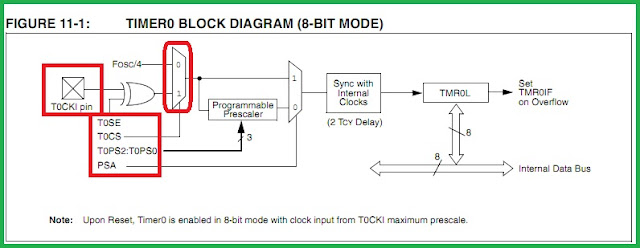IR (Infrared) Remote Controlled Communication Between Two Microcontroller -Step By Step Tutorial : Part-3

Generally We connect crystal clock with microconteroller so that it can work with time. Consider that we are using 12MHz clock. So, that means 12000000 pulses are passing to the microcontroller . Is that clear ?

## Prescalar:

Prescalar is used to measuring frequency. Look at the picture named ratio. When (fosc/4) or (12/4=3MHz) frequency pulse comes to the the microcontroller we need make this smaller to make the calculation easy. So at this stage we need prescalar . Alright!
There is another scaler "Postscalar" . Postscalar works after signal has come to the microcontroller .It isn't very necessary in this tutorial.

On that picture clock cycle  represents (Clock/4) or (12/4=3MHz) frequency . If we use 1:2 to scale , then 2 pulses is considered as 1 pulse . In 1:4 , 4 pulses is considered as one pulse. For 1:8 , eight pulses will be considered as one pulse.

## Working of Timer0

#### Note:To Understand Clearly about Timer0 . You have to keep this picture in your memory . All we see in that part are the details of this Picture and save the Picture .

For Clock frequency we could use T0CKL pin (External Source) but Crystal frequency (12 MHz Crystal Clock) is good for us .

When we connect a crystal (we are using 12MHz Crystal Clock) with our microcontroller , microcotnroller receives pulse continuously. At this case we know the frequency and we can calculate the time period of each pulse from (T=1/f). So if we know how many pulse are received, we can calculate time by multiplying. We will see this later .
Generally , Crystal clock frequency divided by 4. It's a rule. That means first time clock frequency prescaled by 4. Look at the picture and you can see that "clock/4" or "System Clock"IR (Infrared) Communication Between Two Microcontroller -Step By Step Tutorial : Part-3
After this stage signal comes to prescalar. In this tutorial we will use 1:4 prescalar. That means we are dividing the system clock by 4. So, it means when 4 system clock pulse comes to prescalar, it sends out 1 pulse to Timer0 register . In this way timer0 register is being incremented .

Basically, when one pulse enters into the  Timer0 register, TMR0L  register is incremented by 1. The capacity of this register is 0 to 255. So when TMR0L will count 255, there will be an  overflow interrupt. In the interrupt function routine we will reset TMR0L register and we will increase (int count variable) by 1. We need this variable to remember that how many times interrupt occurred. When we perform [count * TMR0L], we get the total number of pulses. From this information we can measure time of "1" and "0"

We will create a project  in Calculation(4th Part)

## Setup Timer0 Register :

Bit 0 to 2 are used to select prescalar. As we are using 1:4 prescalar , we need to set 001

Now set 3rd bit to 0 . So bit comes 0001.

Now set 4th bit to 0 and  we get   00001.

Now set 5th bit to 0 and we get   000001

Now set 6th bit to 1 , because we are using 8 bit mode . You may use 16bit mode. and  we get      1000001

Now set 7th bit to  1, because we need to start timer . Finally we get : 11000001 or 0xc1 (hexadecimal).IR (Infrared) Remote Controlled Communication Between Two Microcontroller -Step By Step Tutorial : Part-3

## Setup Timer0 overflow flag :

When overflow happens timer overflow interrupt flag bit becomes 1. When Overflow Register flag bit becomes 1 and microcontroller understand that interrupt occurred. So microcontroller goes to perform timer interrupt routine. When flag becomes 0 , microcontroller understand that everything is fine and there is no interrupt.
To enable overflow interrupt we need to set that enable. Unless the overflow interrupt will not work.

#### Note: you can find INTCON register on Interrupt section in Datasheet or in Interrupt tutorial i had described about INTCON register.

We set INTCON.TMR0IF bit to 0 and that sets the Flag as 0 .

First we need to enter on the INTCON register and then set TMR0IF=0;

### >>Continue to 4th PART<<

Ain't getting any visitors!

## Featured Post

### Rs 232 , Serial Communication with PIC Microcontroller in Proteus [step by step details]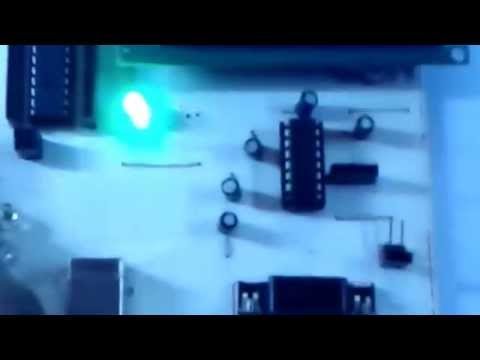## Tags

: (1) 18F2550 (1) 36KHz (3) and (1) arduino (1) Based (1) battery (1) Bipolar (1) Blinking (1) blinks (1) Bluetooth (1) button (1) circuit (1) clock (1) control (1) Db9 (1) DC Motor (2) digital (2) display (2) DS1307 (1) electronic (1) flash (1) flashing (1) HC-06 (1) home (1) how (1) How to (10) i2c tutorial (1) in (1) indicator (1) interface (8) interfacing (3) Interrupt (3) Introduction (1) IR Receiver (4) key pad (1) keyboard (1) keypad (1) lavel (1) Lcd 16x2 (2) lcd 2x16 (2) led (1) lm35 (2) LPG (1) machine (1) make (1) making (1) matrix (1) max232 (1) meter (2) microchip (4) microchips (3) mikroC (5) musical (1) NEC Protocol (4) pcb (5) PIC (3) pic controller (11) pic proteus (1) Pic Tutorial (12) pic18 (2) pic18f2550 (11) picRFモジュール (1) proteus (6) push (1) push button (1) PWM (1) real (1) Rs 232 (1) Rs232 (1) scroll (1) scrolling (1) Serial Port (1) simulation (2) step by step (7) step bystep (1) text (2) time (1) timer (4) timer0 (4) tone (1) tutorial (2) Unipolar (1) USB (1) usb 1.0 (1) USB HID (1) using (9) voltmeter (1) voting (1) with (2) work (1)

## Traffic Feed

Live Traffic Feed
Visitor Tracking